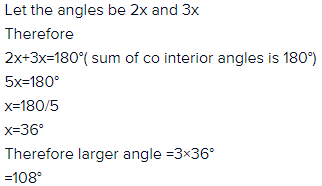Courses

# Test: Parallel Lines And Co-Interior Angles

## 15 Questions MCQ Test Mathematics (Maths) Class 9 | Test: Parallel Lines And Co-Interior Angles

Description
This mock test of Test: Parallel Lines And Co-Interior Angles for Class 9 helps you for every Class 9 entrance exam. This contains 15 Multiple Choice Questions for Class 9 Test: Parallel Lines And Co-Interior Angles (mcq) to study with solutions a complete question bank. The solved questions answers in this Test: Parallel Lines And Co-Interior Angles quiz give you a good mix of easy questions and tough questions. Class 9 students definitely take this Test: Parallel Lines And Co-Interior Angles exercise for a better result in the exam. You can find other Test: Parallel Lines And Co-Interior Angles extra questions, long questions & short questions for Class 9 on EduRev as well by searching above.
QUESTION: 1

### From figure, identify the incorrect statement, given that l || m and t is transversal.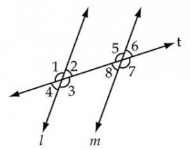Solution:

Angle 2 and 8 are a pair of alternate interior angles, and thus are equal. so they don't form a pair of supplementary angles.

QUESTION: 2

Solution:
QUESTION: 3

### In figure, PQ||RS QPO = 70o ROT = 20o. Find the value of x.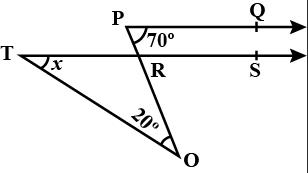Solution:
QUESTION: 4

Given that AB || CD, intersected by a transversal. ∠1 = (120 -x)° and ∠5 = 5x°, then the measures of ∠1 and ∠5 are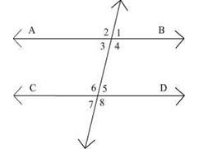Solution: Angle1=angle5(corresponding angles)
therefore,
120-x=5x
or,120=5x+x
or,6x=120
or, x=120/6
or,x=20
and, 120-x=120-20=100
and, 5x=5*20=100

hence proved
QUESTION: 5

l, m and n are parallel lines intersected by a transversal p at X, Y and Z respectively. The value of ∠2 will be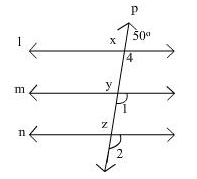Solution:
QUESTION: 6

If AB || CD, CD || EF and y:z = 3:7 then x is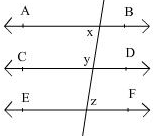Solution:

It is given that,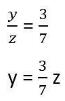Then ∠CON = p

Now CD║EF

So, p = z  (alternate interior angle) .. (1)

Also, p + y = 180°  (linear pair)

y + z = 180° [ from (1) ]

Put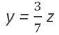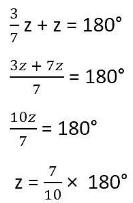z = 126°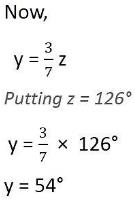Also,  AB║ CD &  MN is transversal. We know that the sum of interior angles on the same side of transversal is supplementry.

x + y = 180°

x + 54° = 180°

x = 180° - 54°

x = 126°

QUESTION: 7

Given that AB || CD, intersected by a transversal, if the complement of ∠5 equals the supplement of ∠4, then the measures of ∠4 and ∠5 are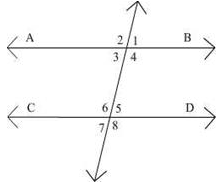Solution:
QUESTION: 8

If a transversal intersects two parallel lines, then the consecutive interior angles are

Solution:
QUESTION: 9

Sum of the measure of an angle and its vertically opposite angle is always.

Solution:
QUESTION: 10

In the figure if AB ||CD, ∠APQ = 50° and ∠PRD = 127° then y is equal to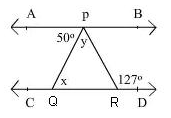Solution: X=50,x+y=127(exterior angle theorem)50+y=127y=127-50y=77
QUESTION: 11

In the given figure, the value of y is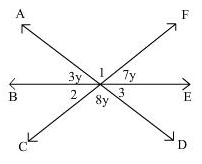Solution:
QUESTION: 12

In the adjoining figure, if PQ || RS, ∠PAB = 45° and ∠ACS = 135°, the value of p and q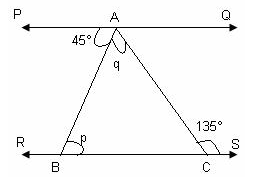Solution:
QUESTION: 13

In the adjoining figure, AB || CD || EF, the value of x is: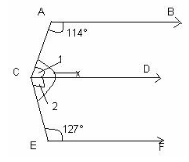Solution:
QUESTION: 14

If two straight lines are perpendicular to a line l, then they are.

Solution:
QUESTION: 15

If two interior angles on the same side of a transversal intersecting two parallel lines in the ratio 2:3 ,then find the larger of two angles.

Solution: